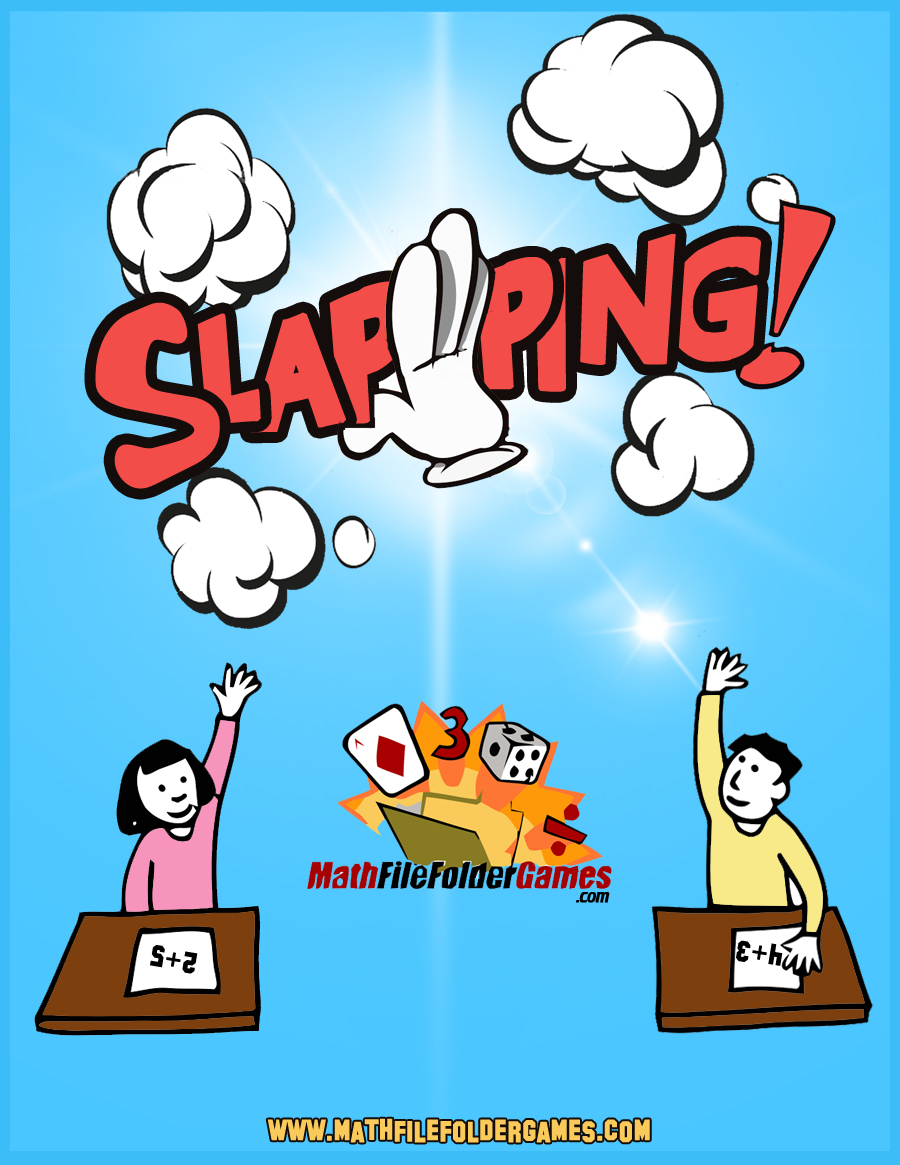# Slapping: Addition & Subtraction Game

##Slapping: Addition & Subtraction Game

• 34 Pages

Buy this Game on TeachersPayTeachers – \$4

#### Rules:

Before play starts the player agree on the sum that will be the key number. For example they might agree that 7 will be the key number.

Addition Key Numbers: 6, 7, 8, 9, 10, 12, 14, 15, 17, 18, 20, 27

The deck is shuffled and completely dealt face-down into two stacks, one on the front of each player. At the same time, both players turn the top cards of their stack face-up, next to each other, in the center of the table. If the sum on one of the cards is the key number, 7 in this example, the players try to slap their hands down on top of that card.

The first player to cover the card with his hand wins the round and collects the face-up cards which he puts at the bottom of his stack. The play ends when one player loses all of his cards or when predetermined time has expired. The player with the greater number of cards is the winner.

#### Variations:

This game can be varied by using subtraction cards or by mixing addition & subtraction
cards.

Subtraction Key Numbers: 6, 7, 8, 9, 10, 12, 14, 15, 17, 18, 20, 27## Operations & Algebraic Thinking

### Represent and solve problems involving addition and subtraction.

• CCSS Math 1.OA.A.1 Use addition and subtraction within 20 to solve word problems involving situations of adding to, taking from, putting together, taking apart, and comparing, with unknowns in all positions, e.g., by using objects, drawings, and equations with a symbol for the unknown number to represent the problem.1
• CCSS Math 1.OA.A.2 Solve word problems that call for addition of three whole numbers whose sum is less than or equal to 20, e.g., by using objects, drawings, and equations with a symbol for the unknown number to represent the problem.

### Understand and apply properties of operations and the relationship between addition and subtraction.

• CCSS Math 1.OA.B.3 Apply properties of operations as strategies to add and subtract.2Examples: If 8 + 3 = 11 is known, then 3 + 8 = 11 is also known. (Commutative property of addition.) To add 2 + 6 + 4, the second two numbers can be added to make a ten, so 2 + 6 + 4 = 2 + 10 = 12. (Associative property of addition.)
• CCSS Math 1.OA.B.4 Understand subtraction as an unknown-addend problem. For example, subtract 10 – 8 by finding the number that makes 10 when added to 8.

### Add and subtract within 20.

• CCSS Math 1.OA.C.5 Relate counting to addition and subtraction (e.g., by counting on 2 to add 2).
• CCSS Math 1.OA.C.6 Add and subtract within 20, demonstrating fluency for addition and subtraction within 10. Use strategies such as counting on; making ten (e.g., 8 + 6 = 8 + 2 + 4 = 10 + 4 = 14); decomposing a number leading to a ten (e.g., 13 – 4 = 13 – 3 – 1 = 10 – 1 = 9); using the relationship between addition and subtraction (e.g., knowing that 8 + 4 = 12, one knows 12 – 8 = 4); and creating equivalent but easier or known sums (e.g., adding 6 + 7 by creating the known equivalent 6 + 6 + 1 = 12 + 1 = 13).

### Work with addition and subtraction equations.

• CCSS Math 1.OA.D.7 Understand the meaning of the equal sign, and determine if equations involving addition and subtraction are true or false. For example, which of the following equations are true and which are false? 6 = 6, 7 = 8 – 1, 5 + 2 = 2 + 5, 4 + 1 = 5 + 2.
• CCSS Math 1.OA.D.8 Determine the unknown whole number in an addition or subtraction equation relating three whole numbers. For example, determine the unknown number that makes the equation true in each of the equations 8 + ? = 11, 5 = _ – 3, 6 + 6 =

This site uses Akismet to reduce spam. Learn how your comment data is processed.